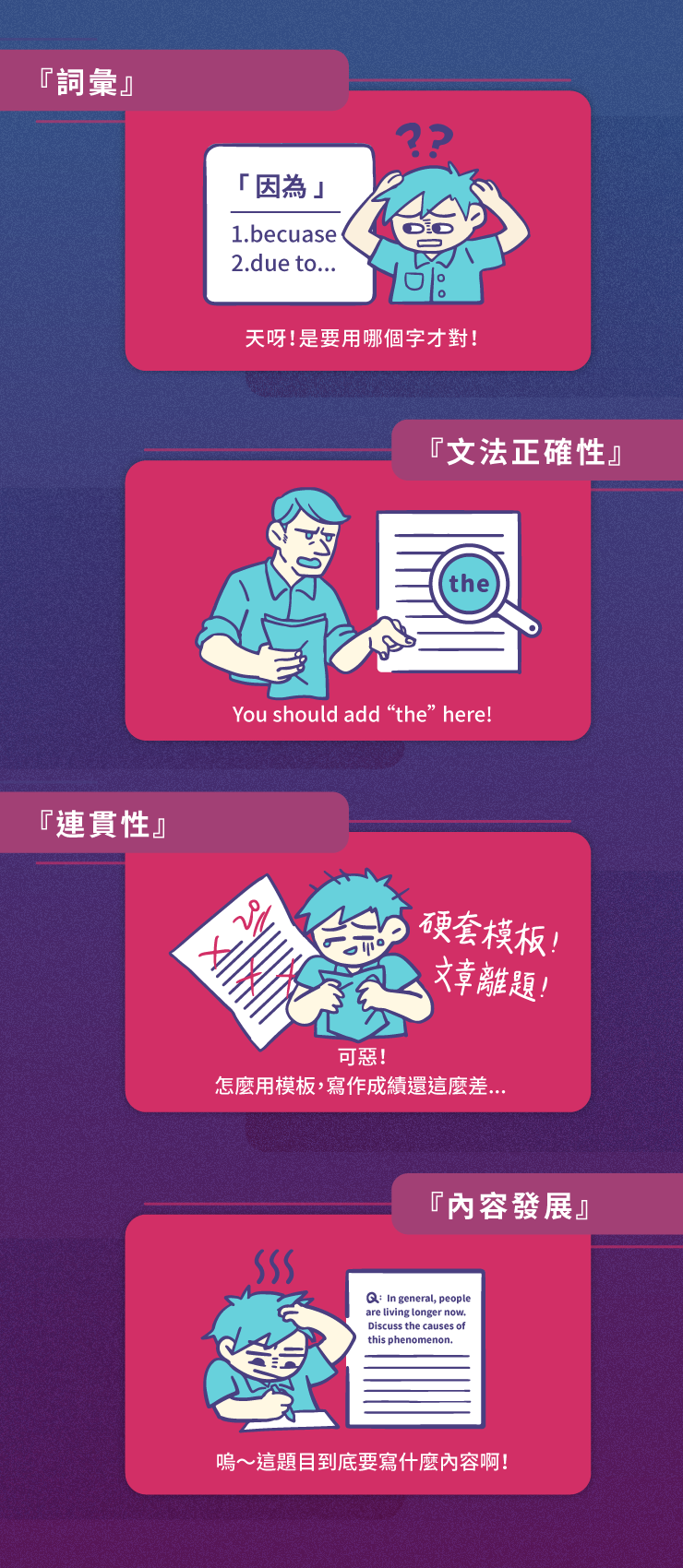＃你以為用「英文」來寫就是英文寫作嗎？

＃你知道什麼是「英文寫作四大元素」嗎？

Books help students learn a lot of knowledge.（不合邏輯、中式英文）

Reading helps students acquire a vast amount of knowledge.（英文邏輯敘述法）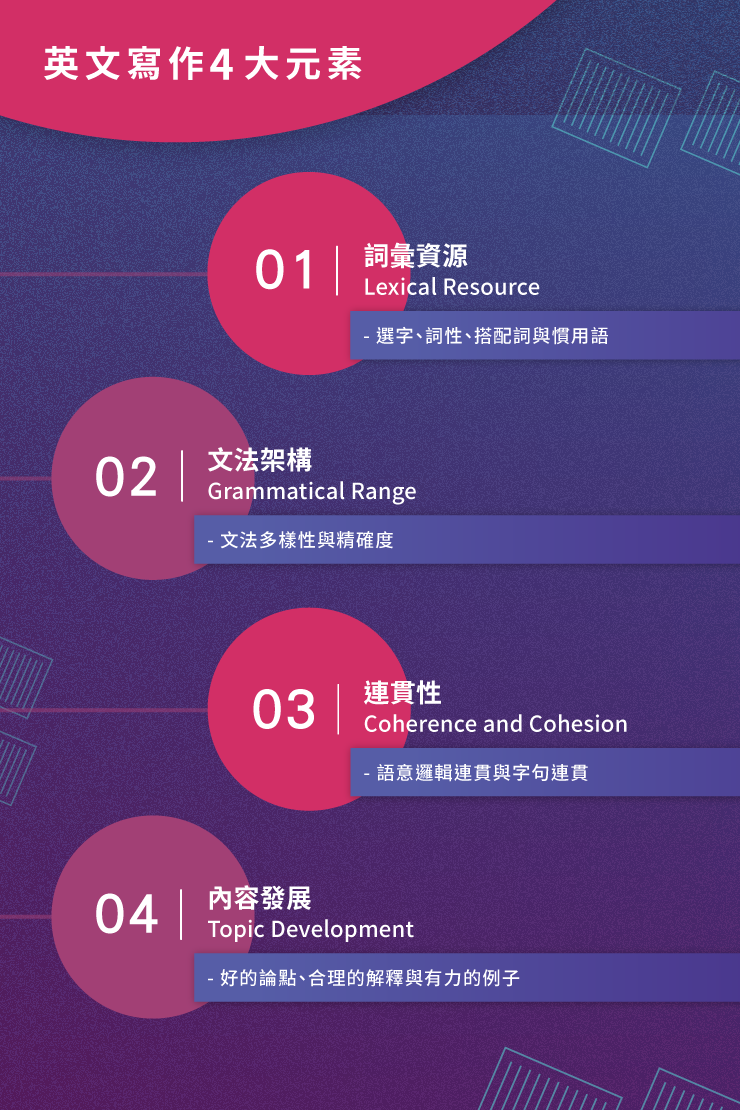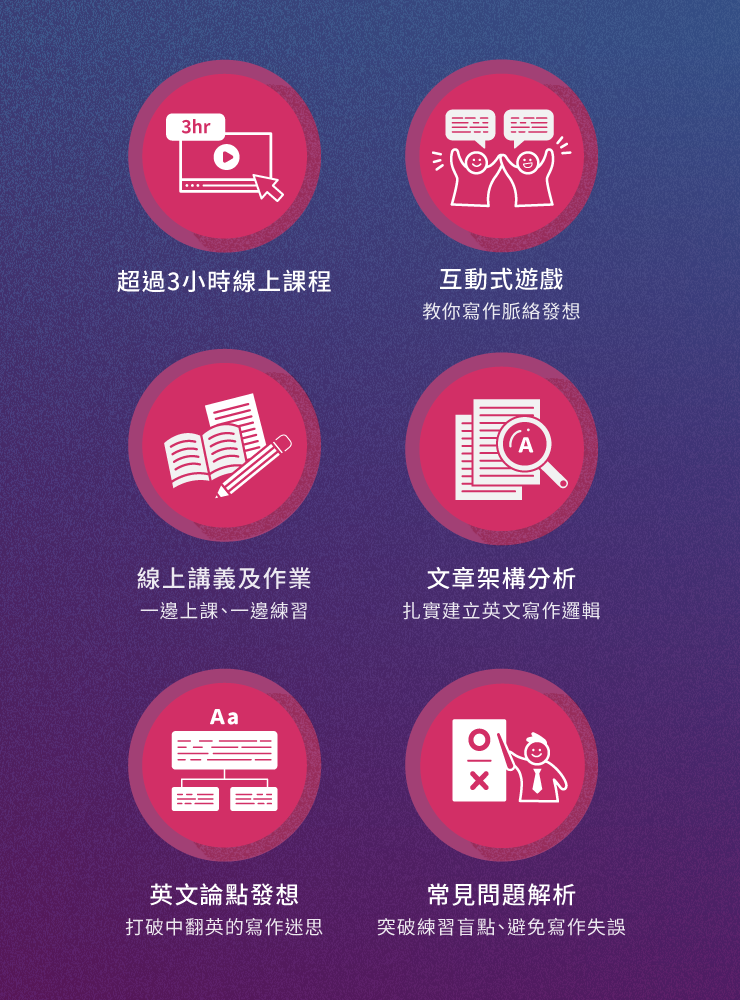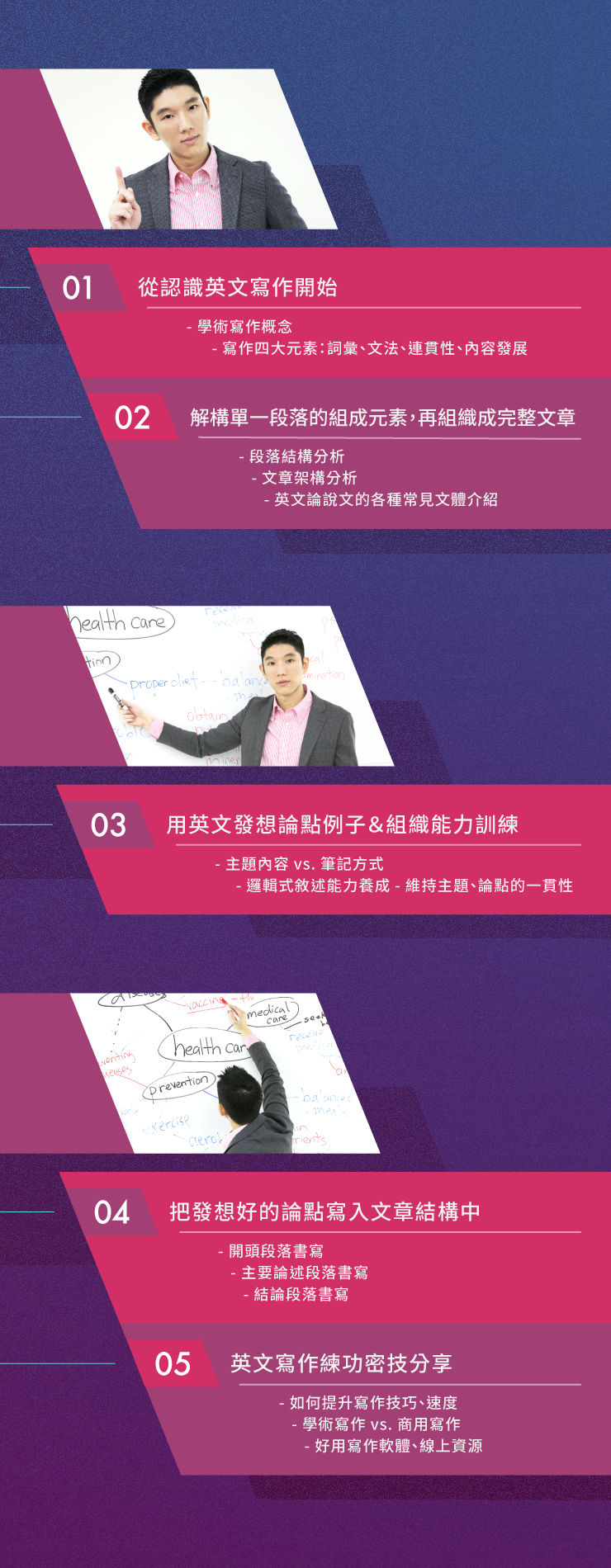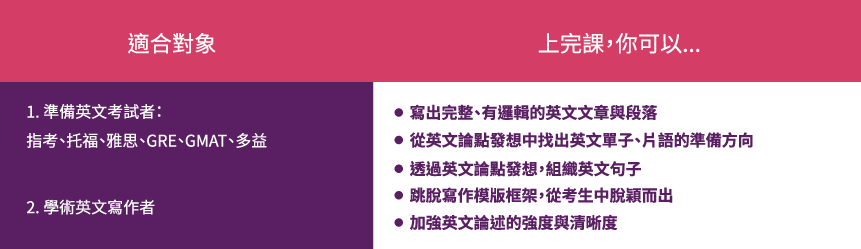3

63分鐘

• 解析英文寫作脈絡＆架構
• 1 英文寫作基本概念｜Introduction to Writing試看 24:21
• 直擊英文寫作各大段落
• 2 英文論點發想｜Prewriting試看 27:39
• 解鎖課程
• 3 推薦英文學習工具｜自動批改、外文字典使用、日常如何增進寫作技巧？試看 11:07

### 開始分享你的學習成果吧！

<%if list.length == 0 %><%else%> <%props list%>

<%if prop.type == 'image' %>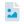圖片檔 <%else prop.type == 'pdf' %>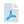PDF <%else prop.type == 'youtube' %>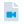Youtube 影片連結 <%else prop.type == 'ppt' %>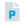PowerPoint <%else prop.type == 'sound' %>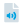錄音檔 <%/if %>

<%:prop.title%>

<%/props%> <%/if%>

### 作業觀摩<%props data%>

<%if prop.type == 'image' %><%else prop.type == 'pdf' %><%else prop.type == 'youtube' %><%else prop.type == 'ppt' %><%else prop.type == 'sound' %><%/if %> 作業<%>~numToZH(~toInt(key) + 1)%> <%:prop.title%>

<%/props%>
<%props data%>
<%if prop.type == 'image'%> <%if prop.cover %>
<%:~isGif(prop.cover)%>
<%else%>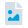<%/if%> <%else prop.type == 'pdf' %>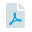<%else prop.type == 'ppt' %>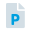<%else prop.type == 'sound' %>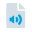<%/if%>
<%if prop.visible == 1 %> <%/if %>

<%:~formatDate(prop.create, 'YYYY-MM-DD')%>

<%:prop.title%>

<%if prop.isAuthor == true %> 刪除 編輯 <%/if %>

<%:prop.comment_count%>

<%/props%>

# 來關注最新的課程資訊吧！<%props data%>

<%:~formatDate(prop.publishDate, 'YYYY/MM/DD')%>

<%:prop.title%>

<%if ~root.isStudent === false && ~root.isTeacher === false && prop.permission === 'private' %>

<%else%> <%:prop.content%> <%/if%>
<%/props%>